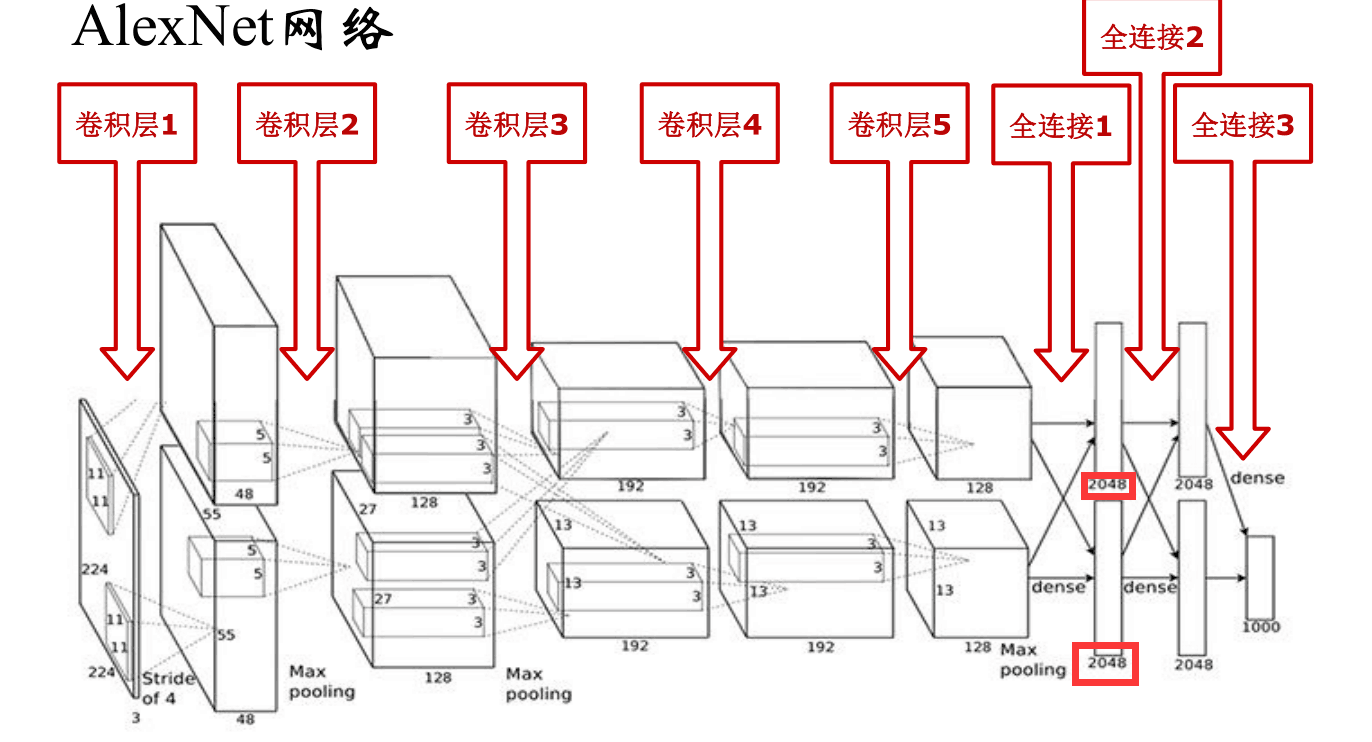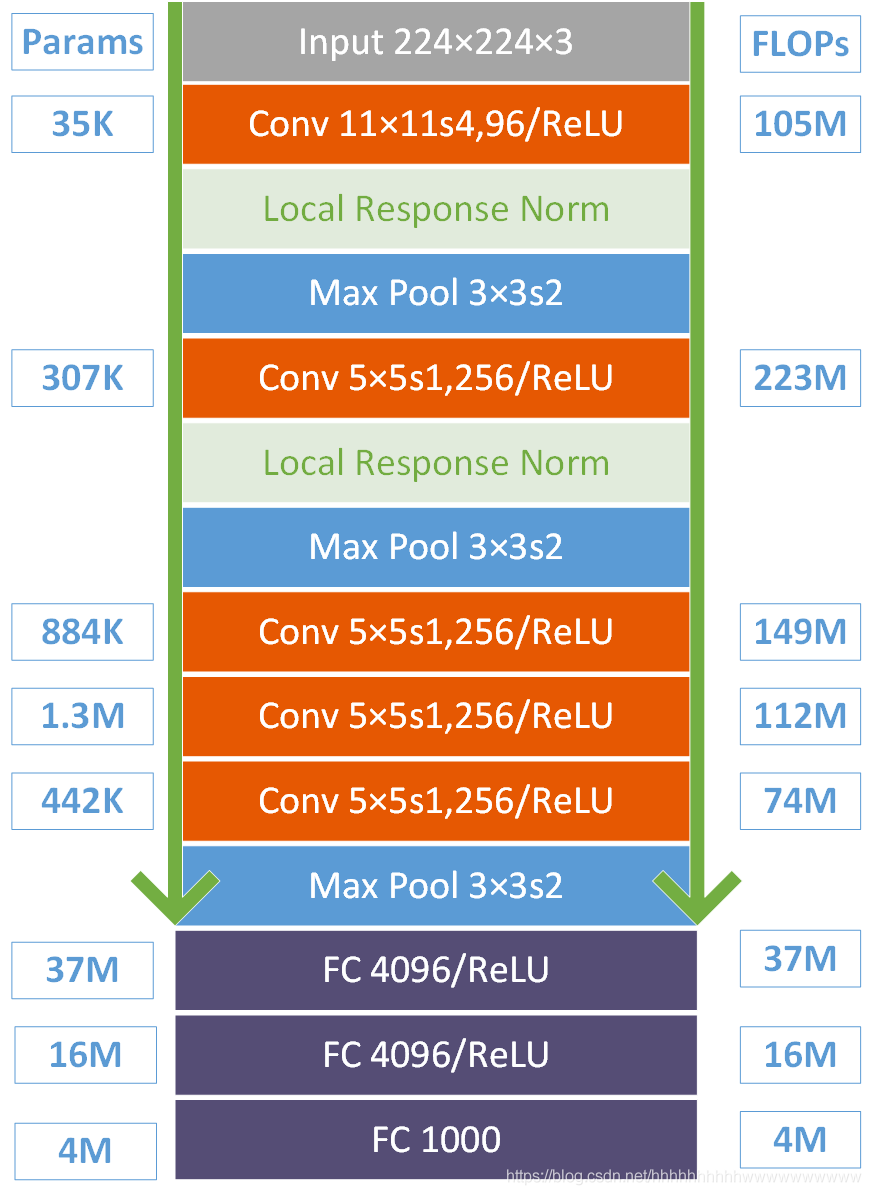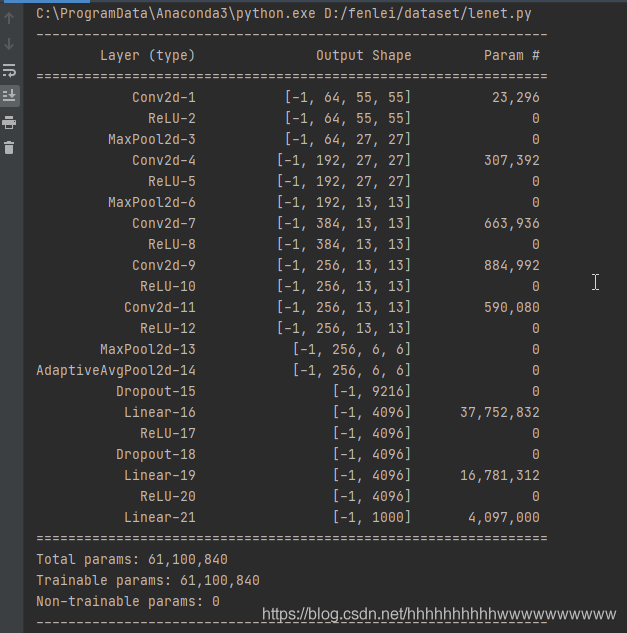【图像分类】 一文读懂AlexNet

+关注继续查看

1、 模型介绍

2、 模型结构

3、 模型特性

4、Pytorch官方实现

5、keras实现

1、 模型介绍

AlexNet是由Alex Krizhevsky 提出的首个应用于图像分类的深层卷积神经网络，该网络在2012年ILSVRC（ImageNet Large Scale Visual Recognition Competition）图像分类竞赛中以15.3%的top-5测试错误率赢得第一名。也是在那年之后，更多的更深的神经网络被提出，比如优秀的vgg,GoogLeNet。 这对于传统的机器学习分类算法而言，已经相当的出色。

AlexNet中包含了几个比较新的技术点，也首次在CNN中成功应用了ReLU、Dropout和LRN等Trick。同时AlexNet也使用了GPU进行运算加速。

AlexNet将LeNet的思想发扬光大，把CNN的基本原理应用到了很深很宽的网络中。AlexNet主要使用到的新技术点如下：

（1）成功使用ReLU作为CNN的激活函数，并验证其效果在较深的网络超过了Sigmoid，成功解决了Sigmoid在网络较深时的梯度弥散问题。虽然ReLU激活函数在很久之前就被提出了，但是直到AlexNet的出现才将其发扬光大。

（2）训练时使用Dropout随机忽略一部分神经元，以避免模型过拟合。Dropout虽有单独的论文论述，但是AlexNet将其实用化，通过实践证实了它的效果。在AlexNet中主要是最后几个全连接层使用了Dropout。

（3）在CNN中使用重叠的最大池化。此前CNN中普遍使用平均池化，AlexNet全部使用最大池化，避免平均池化的模糊化效果。并且AlexNet中提出让步长比池化核的尺寸小，这样池化层的输出之间会有重叠和覆盖，提升了特征的丰富性。

（4）提出了LRN层，对局部神经元的活动创建竞争机制，使得其中响应比较大的值变得相对更大，并抑制其他反馈较小的神经元，增强了模型的泛化能力。

（5）使用CUDA加速深度卷积网络的训练，利用GPU强大的并行计算能力，处理神经网络训练时大量的矩阵运算。AlexNet使用了两块GTX 580 GPU进行训练，单个GTX 580只有3GB显存，这限制了可训练的网络的最大规模。因此作者将AlexNet分布在两个GPU上，在每个GPU的显存中储存一半的神经元的参数。因为GPU之间通信方便，可以互相访问显存，而不需要通过主机内存，所以同时使用多块GPU也是非常高效的。同时，AlexNet的设计让GPU之间的通信只在网络的某些层进行，控制了通信的性能损耗。

（6）数据增强，随机地从256*256的原始图像中截取224*224大小的区域（以及水平翻转的镜像），相当于增加了2*(256-224)^2=2048倍的数据量。如果没有数据增强，仅靠原始的数据量，参数众多的CNN会陷入过拟合中，使用了数据增强后可以大大减轻过拟合，提升泛化能力。进行预测时，则是取图片的四个角加中间共5个位置，并进行左右翻转，一共获得10张图片，对他们进行预测并对10次结果求均值。同时，AlexNet论文中提到了会对图像的RGB数据进行PCA处理，并对主成分做一个标准差为0.1的高斯扰动，增加一些噪声，这个Trick可以让错误率再下降1%。

2、 模型结构wide = (224 + 2 * padding - kernel_size) / stride + 1 = 55

height = (224 + 2 * padding - kernel_size) / stride + 1 = 55

dimention = 963、 模型特性

4、Pytorch官方实现

pytorch的官方并没有严格按照AlexNet论文的实现，第一个卷积是64个卷积核，官方是96个。

class AlexNet(nn.Module):

def __init__(self, num_classes=1000):

super(AlexNet, self).__init__()

self.features = nn.Sequential(

nn.ReLU(inplace=True),

nn.MaxPool2d(kernel_size=3, stride=2),

nn.ReLU(inplace=True),

nn.MaxPool2d(kernel_size=3, stride=2),

nn.ReLU(inplace=True),

nn.ReLU(inplace=True),

nn.ReLU(inplace=True),

nn.MaxPool2d(kernel_size=3, stride=2),

)

self.classifier = nn.Sequential(

nn.Dropout(),

nn.Linear(256 * 6 * 6, 4096),

nn.ReLU(inplace=True),

nn.Dropout(),

nn.Linear(4096, 4096),

nn.ReLU(inplace=True),

nn.Linear(4096, num_classes),

)

def forward(self, x):

x = self.features(x)

x = self.avgpool(x)

x = torch.flatten(x, 1)

x = self.classifier(x)

return x5、keras实现

import os

import pandas as pd

import numpy as np

from keras.callbacks import EarlyStopping, ModelCheckpoint

from matplotlib import pyplot as plt

from skimage import transform

import warnings

from tqdm import tqdm

from keras.layers import Input, Lambda, Conv2D, MaxPool2D, BatchNormalization, Dense, Flatten, Dropout

from keras.models import Model

from keras.utils import to_categorical

def AlexNet(input_shape, num_classes):

inputs = Input(input_shape, name="Input")

x = ZeroPadding2D(((3, 0), (3, 0)))(inputs)

x = Conv2D(96,

(11, 11),

4,

kernel_initializer=initializers.RandomNormal(stddev=0.01),

name="Conv_1")(x)

x = Lambda(tf.nn.local_response_normalization, name="Lrn_1")(x)

x = Activation(activation="relu")(x)

x = MaxPool2D(name="Maxpool_1")(x)

x = Conv2D(256,

(5, 5),

kernel_initializer=initializers.RandomNormal(stddev=0.01),

name="Conv_2")(x)

x = Lambda(tf.nn.local_response_normalization, name="Lrn_2")(x)

x = Activation(activation="relu")(x)

x = MaxPool2D(name="Maxpool_2")(x)

x = Conv2D(384,

(3, 3),

kernel_initializer=initializers.RandomNormal(stddev=0.01),

name="Conv_3_1")(x)

x = Conv2D(384,

(3, 3),

kernel_initializer=initializers.RandomNormal(stddev=0.01),

name="Conv_3_2")(x)

x = Conv2D(256,

(3, 3),

activation="relu",

kernel_initializer=initializers.RandomNormal(stddev=0.01),

name="Conv_3_3")(x)

x = MaxPool2D(name="Maxpool_3")(x)

x = Flatten(name="Flt_1")(x)

x = Dense(4096,

activation="relu",

kernel_initializer=initializers.RandomNormal(stddev=0.01),

name="fc_1")(x)

x = Dropout(0.5, name="drop_1")(x)

x = Dense(4096,

activation="relu",

kernel_initializer=initializers.RandomNormal(stddev=0.01),

name="fc_2")(x)

x = Dropout(0.5, name="drop_2")(x)

output = keras.layers.Dense(num_classes, activation="softmax", name="Output")(x)

m = keras.Model(inputs, output, name="AlexNet")

m.summary()

return m8522 026507 02802 010357 0Xshell使用SSH远程登录阿里云ECS服务器CentOS7
13004 011901 011764 04556 053764 06579 0+关注
390

0

《2021云上架构与运维峰会演讲合集》

《零基础CSS入门教程》

《零基础HTML入门教程》